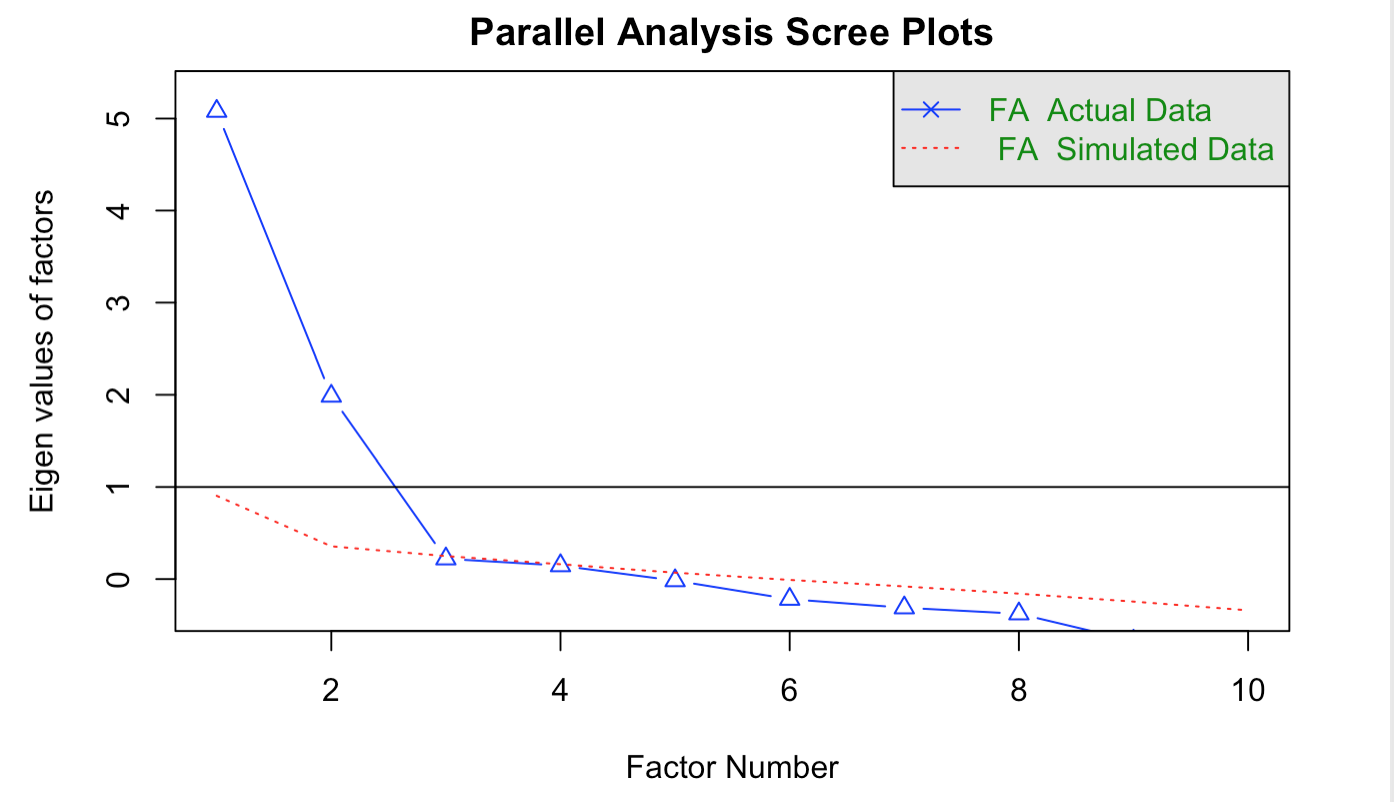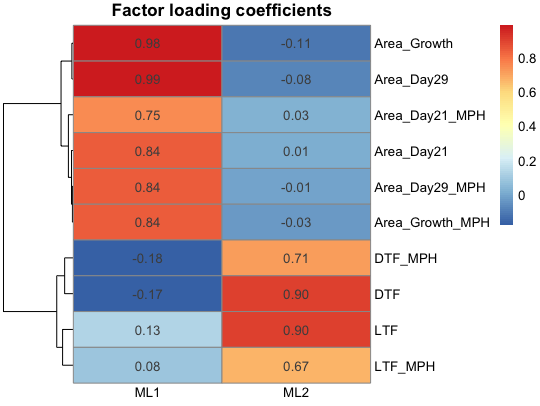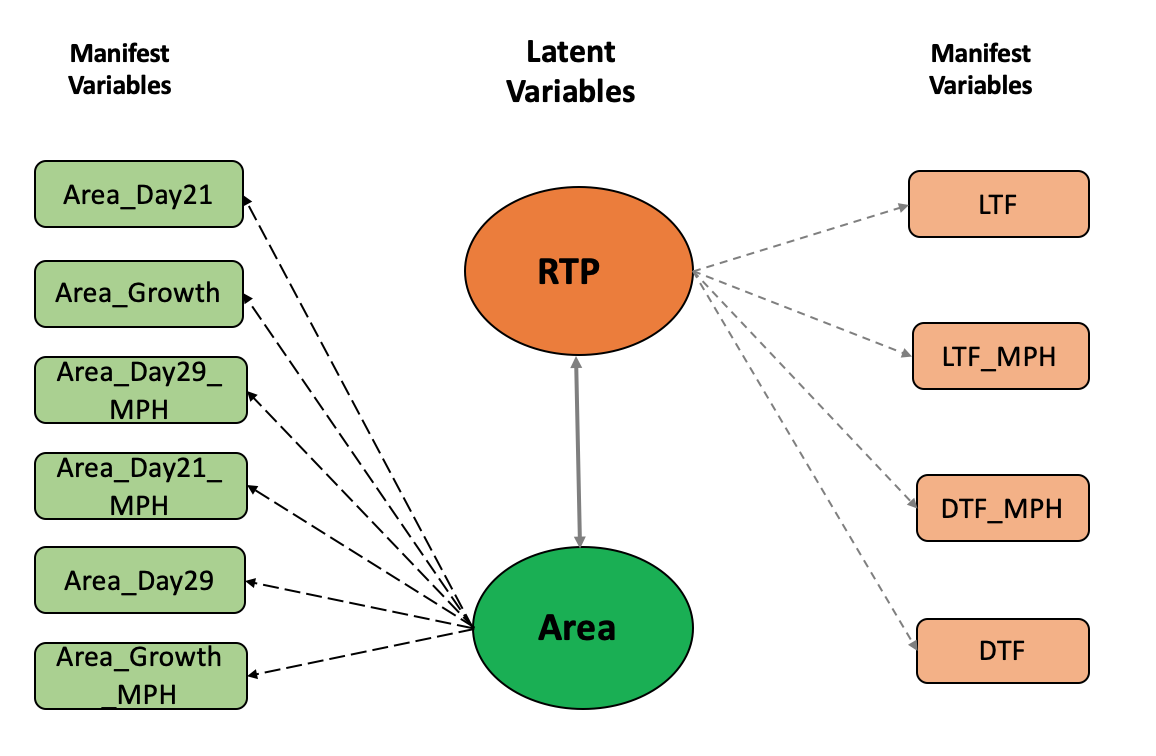# Phenotypic factor analytic model

## Background

Phenotypes are often correlated because of pleiotropy or linkage disequilibrium among QTLs in the complex traits system. A multi-trait mixed model is prevalent in quantiative genetics because it can account for genetic or environmental covariances among phenotypes. Nevertheless, there is a concern of computational efficiency when the number of phenotypes is large, particularly when phenotypes are collected using high-throughput phenotyping platforms.

One alternative approach is to use a factor analytic (FA) model. A FA model assumes that observed phenotypes are generated from underlying unobserved latent variables or factors. In this tutorial, we introduce two types of FA models: exploratory factor analysis (EFA) and confirmatory factor analysis (CFA). We select ten phenotypes related to midparent heterosis or MPH (Area_Day21, Area_Growth, Area_Day29_MPH, Area_Day21_MPH, Area_Day29, Area_Growth_MPH, LTF, LTF_MPH, DTF, and DTF_MPH) from a public Arabidopsis data (Seymour et al. 2016) to illustrate how FA models can be fitted. The Arabidopsis data set used in this tutorial can be found in easyGWAS (Arabidopsis thaliana -> F1 Hybrids Seymour et. al. 2016, PNAS). Alternatively, you can download the data set directly from here using wget or DownGit.

# install.packages("blavaan")
library(blavaan)
# install.packages("pheatmap")
library(pheatmap)
# install.packages("psych")
library(psych)
# install.packages("dplyr")
library(dplyr)
# install.packages("tidyr")
library(tidyr)
# install.packages("knitr")
library(knitr)

## Data import

# Import 10 Arabidopsis phenotypes
dim(pheno) # 372  10
head(pheno)

## Exploratory Factor Analysis (EFA)

When we have no biological prior knowledge regarding the number of factors, EFA can be used to search the underlying factors and infer the latent structure. The EFA is consisted of three steps: 1) identify the number of factors underlying observed phenotypes; 2) fit the EFA model based on the number of factors estimated in 1); and 3) establish the latent structure between factors and phenotypes from factor loading coefficients derived from 2).

### Step 1: Estimate the number of factors

# phenotypic correlation among traits
COR <- cor(pheno, use = "complete.obs")

# parallel analysis to identify the number of underlying factors
fa.parallel(COR, n.obs = 123, fa = "fa", n.iter = 100, error.bars = FALSE,
se.bars = FALSE, ylabel = 'Eigen values of factors', fm = 'mle')The parallel analysis infers the number of factors by comparing the eigenvalues of observed data and the eigenvalues simulated from random data. One criterion to set the number of factors is to count how many eigenvalues from observed data are larger than the eigenvalues from the random data. From the above figure, this number equals two in our example.

### Step 2: Fit EFA model

# fit a maximum likelihood-based EFA model by assuming two factors
fit.mle <- fa(COR, nfactors = 2, fm = "ml", max.iter = 100,
rotate = "varimax")

main = 'Factor loading coefficients', fontsize_number = 10)The factor loading heatmap shows the pattern between the observed phenotypes and factors. We can see that the first factor (ML1) has large factor loading coefficients to the area associated traits, while the second factor (ML2) presents large loadings to the reproductive transition phase (RTP) related traits (i.e., LTF, LTF_MPH, DTF, and DTF_MPH,).

### Step 3: Establish the latent structure

According to the factor loading heatmap, it is possible to eliminate cross-loadings (i.e., phenotype receives factor loadings from more than one factor) by highlighting the highest loading. The inferred latent structure between the observed phenotypes and factors is shown below. We can assign a biological interpretation to those factors: Area and RTP.## Confirmatory factor analysis (CFA)

A CFA model assumes that a latent structure is known. We can fit a CFA model based on the latent structure inferred from EFA.

# CFA model specification
CFA.Model <- '
# 6
Area  =~ Area_Day21 + Area_Growth + Area_Day29_MPH + Area_Day21_MPH +
Area_Day29 + Area_Growth_MPH
# 4
RTP =~ LTF + LTF_MPH + DTF_MPH + DTF
'

# fit the CFA model
CFA.fit <- bcfa(CFA.Model, data = pheno,
burnin = 200, sample = 200, target = "stan",
save.lvs = T, n.chains = 2)

Note that we used a small number of burn-in and Gibbs sampling for an illustrative purpose. In practice, sufficient MCMC samples should be considered and model convergence should be checked.

parameterEstimates(CFA.fit, standardized=TRUE) %>%
filter(op == "=~") %>%
select('Latent Variable' = lhs, 'Observed Phenotypes' = rhs, 'Estimate' = std.all) %>%
kable(digits = 3, format='html', caption ='Standardized Factor Loadings') 
Latent Variable Observed Phenotypes’ Estimate
Area Area_Day21 0.845
Area Area_Growth 0.987
Area Area_Day29_MPH 0.840
Area Area_Day21_MPH 0.752
Area Area_Day29 1.000
Area Area_Growth_MPH 0.835
RTP LTF 0.871
RTP LTF_MPH 0.666
RTP DTF_MPH 0.741
RTP DTF 0.908

### Estimate factor scores

bfs_all <- blavInspect(CFA.fit, 'lvmeans')
colnames(bfs_all) <- c('Area', 'RTP')
dim(bfs_all)
[6,]  -3.666438  0.010334994